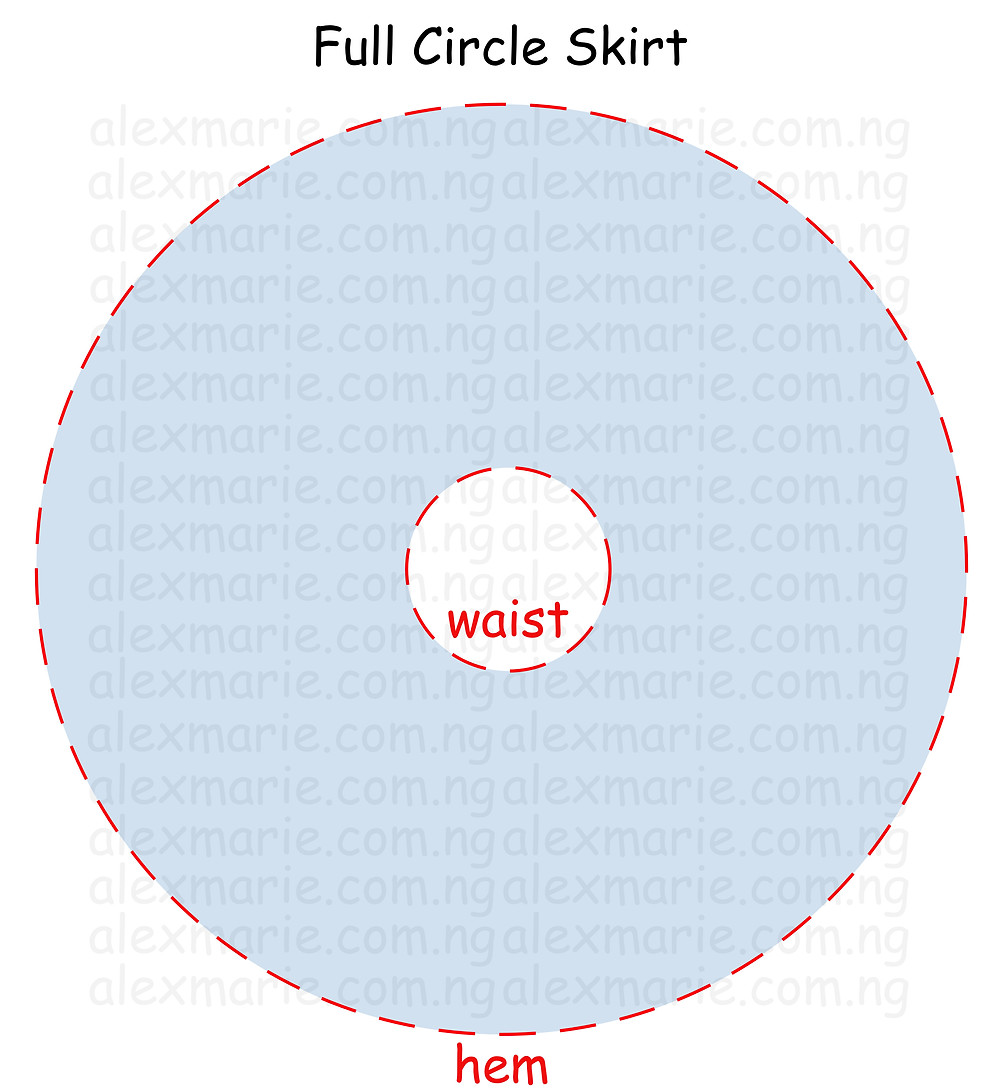Search

# Style Block #3 - All Circle Skirt Variations

Updated: Oct 22, 2021

In this tutorial, we are going to be drafting the classic circle skirt. So called because the waistline and the hemline are circular. This makes the skirt fitted at the waist and full at the hem. The pattern is versatile as it can be used to make not only skirts, but also, tops, flounces, trains, sleeves and even dresses.

## All Circle Skirt Radius FormulasA) Full Circle - r = Waist ÷ 6.28

Mathematical Formula

The Formula for the circumference of a circle is 2πr. In the illustration above, if we assume the Circumference of the Inner circle is the Waist, then the formula would be;

2πr = Waist. The Value of π is 22/7 or 3.14. Therefore 2 x 3.14 x r = Waist.

Now we have to find the value for R i.e. the Radius as the unknown value, because the radius is required to draw a circle (or waist in this case) Therefore;

R = Waist ÷ (2 x 3.14) Or Waist ÷ 6.28

In conclusion the formula for drafting a full Circle Skirt = Waist ÷ 6.28B) Half Circle - r = (Waist x 2) ÷ 6.28

If Half a Circle = Waist, then a Full circle = (Waist x 2) and therefore (Waist x 2) ÷ 6.28=radiusC) Three-quarters Circle - r = (Waist x 1.34) ÷ 6.28

If three-quarters of a Circle = Waist, then a Full circle = (Waist x 1.34) and therefore (Waist x 1.34) ÷ 6.28= radiusD) Double Full Circle - r = (waist ÷ 2) ÷ 6.28

If 2 full Circles = Waist, then 1 full circle = (Waist ÷ 2) and therefore (Waist ÷ 2) ÷ 6.28= radius

### How to Draft a Full Circle1. Use the formula Waist ÷ 6.28 above to calculate the Radius.

2. Placing a tape at the corner (Point 0), mark out the radius from the point going from one edge to the other. This will be the waistline. Label as Points A and B

3. Add seam allowance (0.5inch or 1.2cm) for the waist above the waistline